## 比较下GO、python、delphi、C#、java等执行速度。 热

1：c#，速度还行，4.3秒，这是NET2.0下编译的，不清楚在3.5、4.0、5.0下编译的的执行速度怎么样？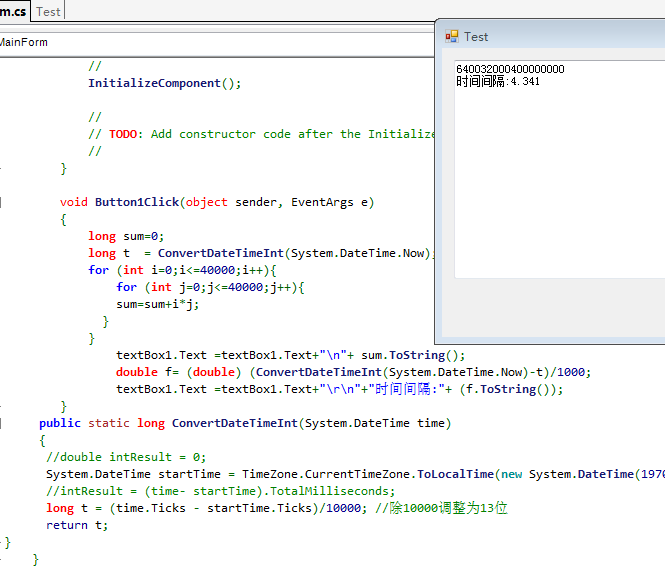2：python，什么啊，需要238秒，知道python的速度慢，但是这个太慢了点，还是用第三方库文件来计算吧。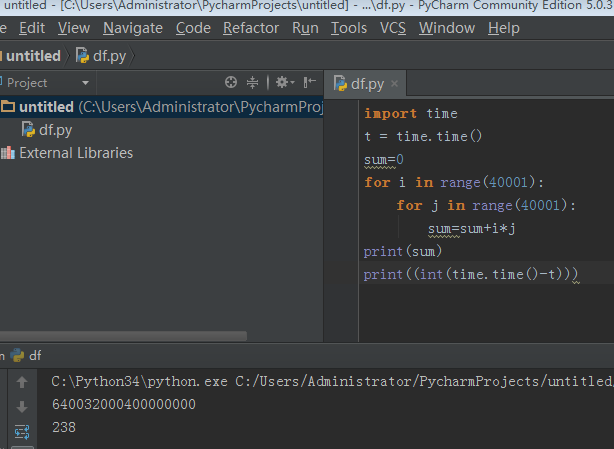3：我们中国的中文编程语言 ，易语言，20秒的样子，不上不下。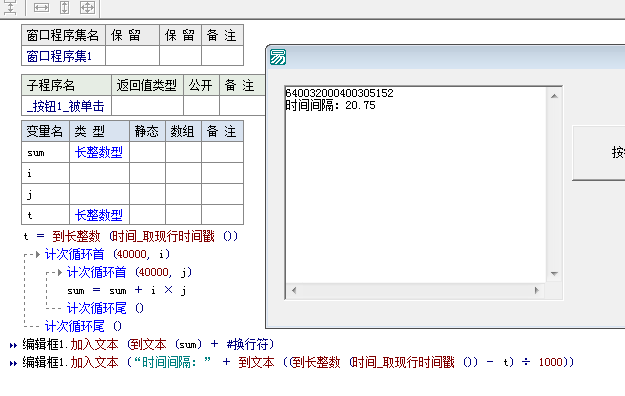4：delphi 这是一门强大的语言，只不过用D7来编译，好像3.53秒的样子。D7本是WIN32位下面的产物，现在速度慢了点也无关紧要。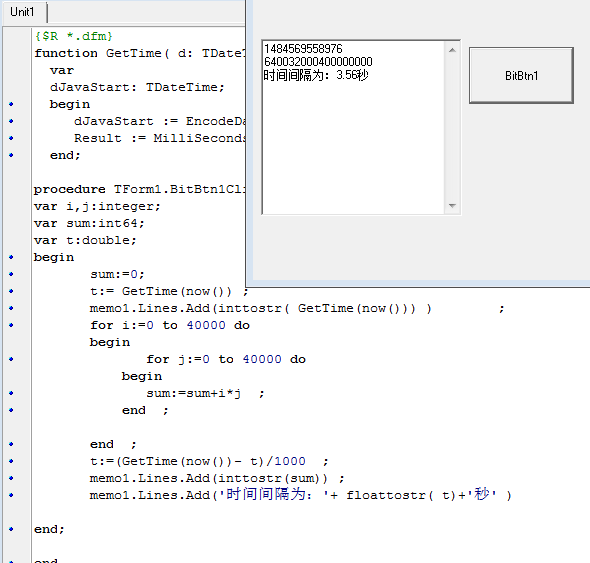5：JAVA,2.63秒的速度，好像并不慢嘛，应该是JAVA根据平台优化的结果。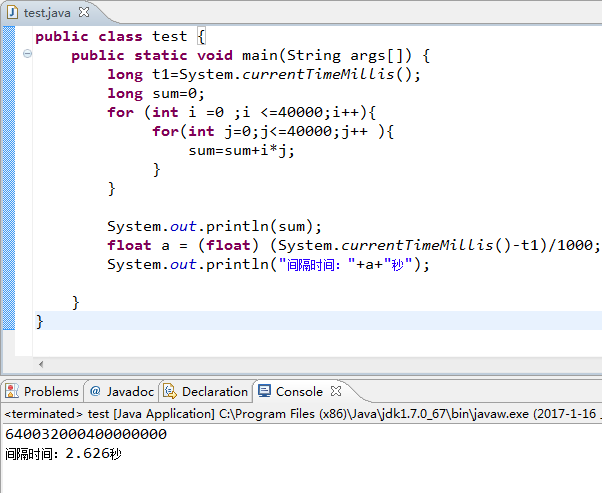6：GO语言，速度这么快？？？GO1.74编译的结果。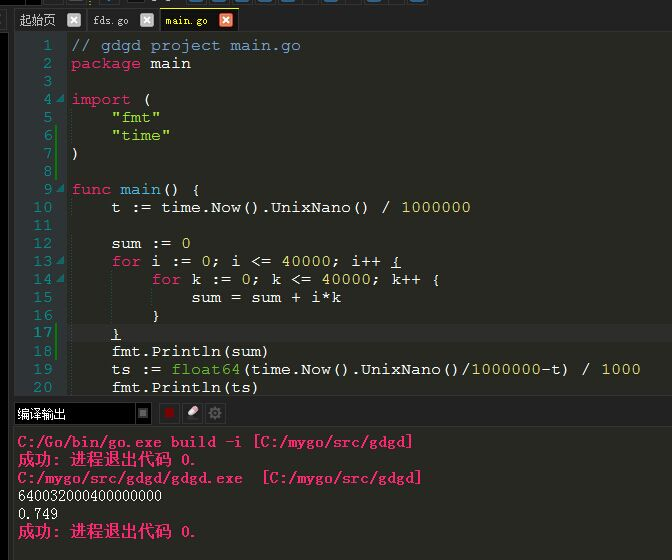2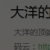7：PHP，根据下面的yak修改，需要66秒。排名比python强，在PHP5.4下测试。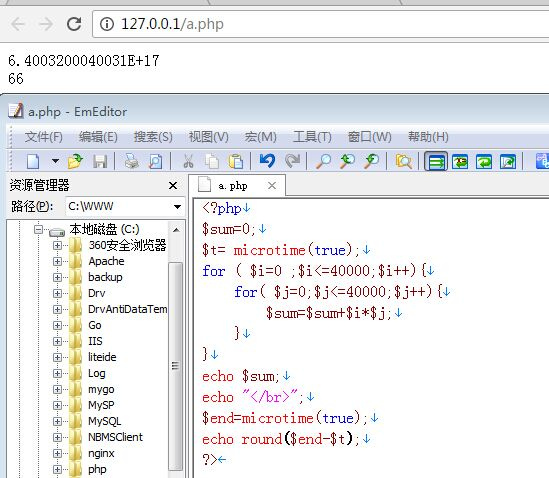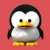2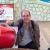1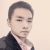1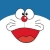1
M

1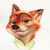```#include <stdio.h>
#include <stdlib.h>
#include <stdint.h>
#include <time.h>

int
main(int argc, char *argv[])
{
time_t t1 = time(NULL);
uint64_t sum = 0, i, j;
for(i = 0; i <= 40000; ++i) {
for(j = 0; j <= 40000; ++j) {
sum += i * j;
}
}
time_t t2 = time(NULL);
printf("result : %lu", sum);
printf("spend time : %u", (uint32_t)(t2 - t1));
return 0;
}```

1```import time
from functools import reduce

t = time.time()
sum = 0
def loop_i_j():
for i in range(40001):
for j in range(40001):
yield i * j
sum = reduce(lambda a, b : a + b, loop_i_j())
print(sum)
print(time.time() - t)```

root @host :~/pypy3-v5.5.0-linux64/bin# ./pypy3 /root/t_spd.py
640032000400000000
11.952942371368408